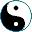Haskell introduced IO monad as if to overcome the awkwardness of explanation, how come a function can have a side-effect. Here is the trick they use in Haskell. Take the State Machine Monad, as we did before. Imagine that A, the state set, represents the whole outside world, and X is the set of function results. In this model, every state machine becomes a function. A is further represented as a cartesian product of two String sets, the first component being "input", and the second - "output". Special transition functions are introduced, one, `getc`, pops a character from input; the other, `putc`, adds a character to output. I am just curious, what exactly does this model try to achieve.